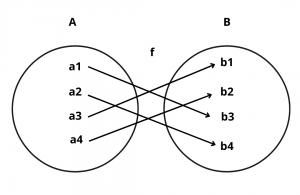# One One and Onto Function (Bijection) – Definition and Examples

Here, you will learn one one and onto function (bijection) with definition and examples.

Let’s begin –

## What is Bijection Function (One-One Onto Function) ?

Definition : A function f : A $$\rightarrow$$ B is a bijection if it is one-one as well as onto.

In other words, a function f : A $$\rightarrow$$ B is a bijection, if it is

(i) one-one i.e. f(x) = f(y) $$\implies$$ x = y for all x, y $$\in$$ A.

(ii) onto i.e. for all y $$\in$$ B, there exist x $$\in$$ A such that f(x) = y.

Example : Let f : A $$\rightarrow$$ B be a function represented by the following diagram :Solution : Clearly, f is a bijection since it is both one-one (injective) and onto (surjective).

Example : Prove that the function f : Q $$\rightarrow$$ Q given by f(x) = 2x – 3 for all x $$\in$$ Q is a bijection.

Solution : We observe the following properties of f.

One-One (Injective) : Let x, y be two arbitrary elements in Q. Then,

f(x) = f(y) $$\implies$$ 2x – 3 = 2y – 3 $$\implies$$ 2x = 2y $$\implies$$ x = y

Thus, f(x) = f(y) $$\implies$$ x = y for all x, y $$\in$$ Q.

So, f is one-one.

Onto (Surjective) : Let y be an arbitrary element of Q. Then,

f(x) = y $$\implies$$ 2x – 3 = y $$\implies$$ x = $$y + 3\over 2$$

Clearly, for all y $$\in$$ Q, x = $$y + 3\over 2$$ $$\in$$ Q. Thus, for all y $$\in$$ Q (co-domain) there exist x $$\in$$ Q (domain) given by x = $$y + 3\over 2$$ such that f(x) = f($$y + 3\over 2$$) = 2($$y + 3\over 2$$) – 3 = y. That is every element in the co-domain has its pre-image in x.

So, f is onto function.

Hence, f : Q $$\rightarrow$$ Q is a bijection.

Example : Let f : R $$\rightarrow$$ R be a function defined as f(x) = $$2x^3 + 6x^2$$ + 12x +3cosx – 4sinx; then f is-

Solution : We have f(x) = $$2x^3 – 6x^2$$ + 12x + 3cosx – 4sinx

$$\implies$$ f'(x) = $$6x^2 – 12x$$ + 12 – 3sinx – 4cosx

$$\implies$$ $$6x^2 – 12x$$ + 12 = 6($$x-1)^2$$ + 6 = g(x) and 3sinx + 4cosx = h(x)

range of g(x) = [6, $$\infty$$)

range of h(x) = [-5, 5]

hence f'(x) always lies in the interval [1, $$\infty$$)

$$\implies$$ f'(x) > 0

Hence f(x) is increasing i.e. one-one

Now x $$\rightarrow$$ $$\infty$$ $$\implies$$ f $$\rightarrow$$ $$\infty$$ & x $$\rightarrow$$ -$$\infty$$ $$\implies$$ f $$\rightarrow$$ -$$\infty$$ & f(x) is continous.

hence its range is R $$\implies$$ f is onto so f is bijective.

Note :

(i)  If a line parallel to x-axis cuts the graph of the functions atmost at one point, then the f is one-one.

(ii)  If any line parallel to x-axis cuts the graph of the functions atleast at two points, then f is many-one.

(iii)  If continous functions f(x) is always increasing or decreasing in whole domain, then f(x) is one-one.

(iv)  All linear functions are one-one.

(v)  All trigonometric functions in their domain are many one.

(vi)  All even degree polynomials are many one.

(vii)  Linear by linear is one-one.

(ix)   A polynomial function of degree even define from R $$\rightarrow$$ R will always be into.

(x)  A polynomial function of degree odd defined from R $$\rightarrow$$ R will always be onto

(xi)  Quadratic by quadratic without any common factor define from R $$\rightarrow$$ R is always an into function.

Thus a function can be of these four types :

(i)  one-one onto (Injective and Surjective)(Also known as Bijective mapping)

(ii)  one-one into (Injective but not surjective)

(iii)  many-one onto (surjective but not injective)

(iv)  many-one into (neither surjective nor injective)